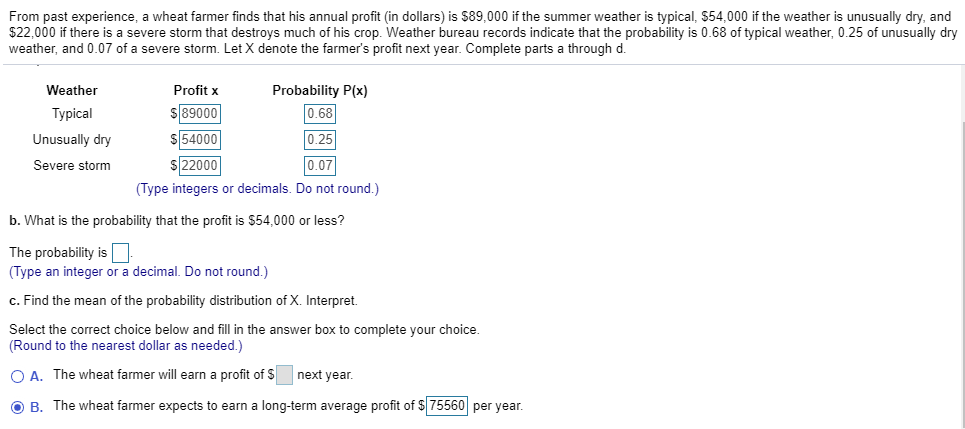# From past experience, a wheat farmer finds that his annual profit (in dollars) is \$89,000 if the summer weather is typical, \$54,000 if the weather is unusually dry, and\$22,000 if there is a severe storm that destroys much of his crop. Weather bureau records indicate that the probability is 0.68 of typical weather, 0.25 of unusuallly dryweather, and 0.07 of a severe storm. Let X denote the farmer's profit next year. Complete parts a through d.Probability P(x)WeatherProfit x\$89000\$54000\$220000.68Typical0.25Unusually dry0.07Severe storm(Type integers or decimals. Do not round.)b. What is the probability that the profit is \$54,000 or less?The probability is(Type an integer or a decimal. Do not round.)c. Find the mean of the probability distribution of X. Interpret.Select the correct choice below and fill in the answer box to complete your choice.(Round to the nearest dollar as needed.)OA. The wheat farmer will earn a profit of Snext yearO B. The wheat farmer expects to earn a long-term average profit of \$75560 per year.

Question
78 views

How would you find \$54000 or less?help_outlineImage TranscriptioncloseFrom past experience, a wheat farmer finds that his annual profit (in dollars) is \$89,000 if the summer weather is typical, \$54,000 if the weather is unusually dry, and \$22,000 if there is a severe storm that destroys much of his crop. Weather bureau records indicate that the probability is 0.68 of typical weather, 0.25 of unusuallly dry weather, and 0.07 of a severe storm. Let X denote the farmer's profit next year. Complete parts a through d. Probability P(x) Weather Profit x \$89000 \$54000 \$22000 0.68 Typical 0.25 Unusually dry 0.07 Severe storm (Type integers or decimals. Do not round.) b. What is the probability that the profit is \$54,000 or less? The probability is (Type an integer or a decimal. Do not round.) c. Find the mean of the probability distribution of X. Interpret. Select the correct choice below and fill in the answer box to complete your choice. (Round to the nearest dollar as needed.) OA. The wheat farmer will earn a profit of Snext year O B. The wheat farmer expects to earn a long-term average profit of \$75560 per year. fullscreen
check_circle

Step 1

Probability:

Probability of an event is the number of outcomes favourable to the event, divided by the total number of possible outcomes in the study.

Suppose the event of interest is A. If the number of cases favou...

### Want to see the full answer?

See Solution

#### Want to see this answer and more?

Solutions are written by subject experts who are available 24/7. Questions are typically answered within 1 hour.*

See Solution
*Response times may vary by subject and question.
Tagged in

### Statistics